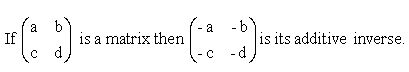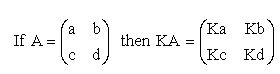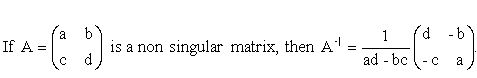kwizNET Subscribers, please login to turn off the Ads!
 Email us to get an instant 20% discount on highly effective K-12 Math & English kwizNET Programs!

#### Online Quiz (WorksheetABCD)

Questions Per Quiz = 2 4 6 8 10

### High School Mathematics - 26.4 Important Points in Matrices

 1. A matrix is a rectangular array or elements arranged in rows and columns. 2. If a matrix has m rows and n columns, we say that it is an m � n matrix. The order of the matrix is m � n. 3. If m � n, then we call it a rectangular matrix. 4. If m = n, then we call it a square matrix. 5. A matrix that has only one row is called a row matrix. A matrix that has only one column is called a column matrix. 6. The matrix in which every element is zero is called a zero matrix or null matrix and it is denoted by the capital letter "O". 7.The diagonal from the first element of the first row to the last element of the last row is called the Principal diagonal. A square matrix in which all the elements in the principal diagonal are equal and the rest of the elements are zeros is called a scalar matrix. 8. A square matrix in which each element of the principal diagonal is '1' and the other elements are zero is called an identity matrix or unit matrix, and is denoted by 'I'. An identity matrix is a scalar matrix also. 9. If A is a matrix, the matrix obtained by interchanging the rows and columns of A is called its transpose and is denoted by AT. 10. Two matrices A and B are said to be equal if and only if they are of the same order and their corresponding sides are equal. 11. The zero matrix is the identity element in matrix addition. i.e., A + O = O + A = A. 12. Matrix addition is commutative. i.e., A + B = B + A. 13. Matrix addition is associative. i.e., (A+B)+C = A+(B+C). 14.15. A-B is defined as A+(-B). 16. If K, l are real number then, K(A+B) = KA + KB; (K+l)A = KA + lA. 17. If A and B are two matrices, then their product AB is defined only when the number of columns in A is equal to the number of rows in B. Then A and B are said to be conformable for multiplication. 18. In general the multiplication of matrices not commutative. 19.20. Multiplication of matrices is associative. i.e., (AB)C = A(BC). 21. If I is the unit matrix then AI = IA = A, I is the density element in matrix multiplication. 22. If AB = 0, then it is not necessary that atleast A or B is a zero matrix. 23. a(B+C) = AB + BA and (B+C)A = BA + CA, distributive law. 24. If the transpose of a given matrix is equal to its additive inverse, then the matrix is called skew symmetric matrix. 25. The determinant of A is denoted by |A| or Det A. 26. If the determinant of matrix is zero it is called a singular matrix. 27. If the determinant of matrix is not zero it is called a non-singular matrix. 28. If A is a non-singular matrix and B is another matrix such that Ab = BA = I, then B is called the inverse of A and is denoted by A-|. If AB = BA = I, then B = A-|. If A-|, then B-|. 29.30. (AB)-| = B-|A-|. 31. If A, X and B are matrices such that AX = B, then X = A-|B. Directions: Choose the correct answer.
 Q 1: For the system of equations AX = B, X is called the _______ matrix.variablecoefficientconstant Q 2: If a matrix is equal to its transpose, i.e., A = AT it is called a _____ matrix.skew symmetricinversetransposesymmetric Q 3: The matrix ATthat is obtained by interchanging the rows and columns of a given matrix A is called _____ matrix.inversenone of thesetransposesymmetric Q 4: For the system of equations AX = B, A is called the _______ matrix.variablecoefficientconstant Q 5: Two matrices A and B are said to be equal it and only if they are of _____ order.samedifferent Q 6: A matrix in which each element is zero is called _______.bothnull matrixzero matrix Question 7: This question is available to subscribers only! Question 8: This question is available to subscribers only!

#### Subscription to kwizNET Learning System offers the following benefits:

• Unrestricted access to grade appropriate lessons, quizzes, & printable worksheets
• Instant scoring of online quizzes
• Progress tracking and award certificates to keep your student motivated
• Unlimited practice with auto-generated 'WIZ MATH' quizzes
• Child-friendly website with no advertisements
• Choice of Math, English, Science, & Social Studies Curriculums
• Excellent value for K-12 and ACT, SAT, & TOEFL Test Preparation
• Get discount offers by sending an email to discounts@kwiznet.com

 Quiz Timer